# Covariance

A numerical characteristic of the joint distribution of two random variables, equal to the mathematical expectation of the product of the deviations of these two random variables from their mathematical expectations. The covariance is defined for random variables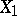and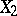with finite variance and is usually denoted by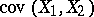. Thus,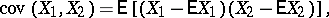so that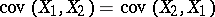;. The covariance naturally occurs in the expression for the variance of the sum of two random variables: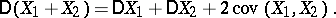Ifandare independent random variables, then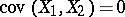. The covariance gives a characterization of the dependence of the random variables; the correlation coefficient is defined by means of the covariance. In order to statistically estimate the covariance one uses the sample covariance, computed from the formulawhere the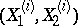,, are independent random variables and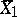and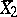are their arithmetic means.

In the Western literature one always uses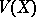or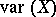for the variance, instead of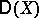.